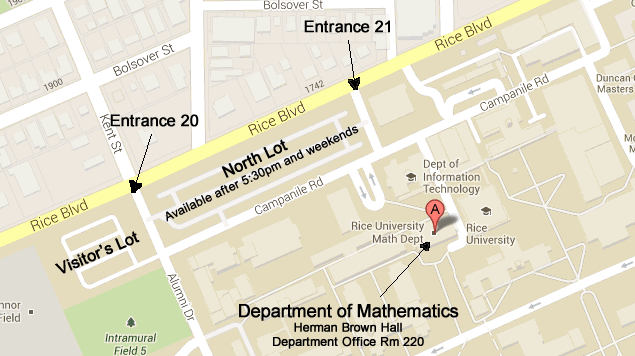## 2021 Ph.D Thesis Defenses

#### Chengcheng Yang

Title: Properties of Shortest Length Curves inside Semi-algebraic Sets and Problems related to an Erdös Conjecture concerning Lattice Cubes

Part I: Properties of Shortest Length Curves inside Semi-algebraic Sets

This part concerns an analytical stratification question of real algebraic and semi- algebraic sets. In 1957 Whitney  gave a stratification of real algebraic sets, it partitions a real algebraic set into partial algebraic manifolds. In 1975 Hironaka  reproved that a real algebraic set is triangulable and also generalized it to sub-analytic sets, following the idea of Lojasiewicz’s  triangulation of semi-analytic sets in 1964. During the same year, Hardt  also proved the triangulation result for sub-analytic sets by inventing an- other method. Since any semi-algebraic set is also semi-analytic, thus is sub-analytic, both Hironaka and Hardt’s results showed that any semi-algebraic set is homeomorphic to the polyhedron of some simplicial complex.

Following their examples and wondering how geometry looks like locally for a semi- algebraic set, Part I of the paper tries to come up with a stratification, in particular a cell decomposition, such that it satisfies the following analytical property. Given any arbitrary semi-algebraic set X in R2, any two points in X may be joined by a continuous path γ of shortest length. We will show that there exists a semi-algebraic cell decomposition A of X such that for each A ∈ A, each component of γ ∩ A is either a singleton or a real analytic geodesic segment in A; furthermore, γ ∩ A has at most finitely many components.

Part II: Problems related to an Erd¨os conjecture concerning lattice cubes.

Part II of the paper studies a problem of Erd¨os concerning lattice cubes. Given an N × N × N lattice cube, we want to find the maximum number of vertices one can select so that no eight corners of a rectangular box are chosen simultaneously. Erd¨os conjectured that it has a sharp upper bound, which is O(N 11/4), but no example that large has been found yet. We start approaching this question for small N using the method of exhaustion, and we find that there is not necessarily a unique maximal set of vertices (counting all possible symmetries). Next, we study an equivalent two-dimensional version of this problem looking for patterns that might be useful for generalizing to the three-dimensional case. Since an n×n grid is also an n×n matrix, we rephrase and generalize the original question to: what is the minimum number α(k, n) of vertices one can put in an n × n matrix with entries 0 and 1, such that every k × k minor contains at least one entry of 1, for 1 ≤ k ≤ n? We discover some interesting formulas and asymptotic patterns that shed new light on the question. Then we examine many examples that succeed for O(n8/3) but fail for O(n11/4).
Last we try to prove by contradiction that Erd¨os’ conjecture is wrong, and that O(n8/3) is the sharp upper bound for the maximum number. Here we work not only with discrete cases using combinatorics, but also with continuous cases, such as topological manifolds, using linear groups.Large Map

Tel (713) 348-4829